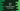# Java example to find missing number in an array of sequenceIn this Java programming tutorial, we will learn how to find a missing number in an array of continuous numbers.The numbers in the array will be shuffled.For example, for the numbers 1,2,3,5, we know that 4 is missing. Our program will find out this value. In the example below, we will try two different approaches : one to find out one single missing number and the second one to find out multiple missing numbers. Let’s take a look at the examples below :

### Java example to find one missing number in an array of sequence of numbers,if maximum number of the series is given:

``````/**
* Class to find missing number in an array
*/
public class Test {

static void print(String value) {
System.out.print(value);
}

/**
* Function to find the missing number in a array
* @param arr : given array
* @param max : value of maximum number in the series
*/
static void findMissingNumber(int[] arr, int max){
//3
int sumOfAllNumbers = 0;
int currentSum = 0;

//4
//find the sum of all numbers
sumOfAllNumbers = (max * (max + 1))/2;

//5
//find sum of all elements of the array by iterating through them
for (int i =0;i<arr.length;i++){
currentSum += arr[i];
}

print("Missing number "+ (sumOfAllNumbers - currentSum));

}

public static void main(String[] args) {
//1
int maxNumber = 10;
int[] givenArr = {1,2,3,5,7,9,6,8,10};

//2
findMissingNumber(givenArr, maxNumber);

}
}``````

### Explanation :

The commented numbers in the above program denote the step number below :

1. In this example, maximum number of the sequence is given.If the maximum number is not available, we can easily find it using one loop.Store the maximum number in maxNumber variable and store the number sequence in givenArr variable.
2. Call the findMissingNumber method to find out the missing number in the sequence.
3. To find the missing number, we will find out the sum of all numbers in the sequence including the missing number and sum of all current numbers of the sequence. The missing number will be the difference between these sum.
4. Find the sum of all numbers including the missing number and store it in sumOfAllNumbers variable.
5. Find the sum of all current numbers and store it in the currentSum variable.
6. Find the difference and print out the result. This should be the missing number.

### Find multiple missing numbers in a sequence array:

Suppose, we have multiple numbers are missing in the sequence.For example, in 1,3,6,7 sequence, we have 2,4,5 missing. First we will sort the array .Then to find these numbers, we will use one loop. If any number is found missing, we will use one more inner loop to print all numbers starting from that missing number that are not in the list.Let’s take a look at the program :

### Java program :

``````import java.util.Arrays;

/**
* Class to find missing number in an array
*/
public class Test {

static void print(String value) {
System.out.println(value);
}

/**
* Function to find the missing number in a array
*
* @param arr : given array
* @param max : value of maximum number in the series
*/
static void findMissingNumber(int[] arr, int max) {

//3
Arrays.sort(arr);
//4
int currentValue = 1;

//5
for (int i = 0; i < arr.length; i++) {
//6
if (arr[i] != currentValue) {
for (int j = currentValue; j < arr[i]; j++) {
print("Missing number Found : " + j);
}
}
currentValue = arr[i] + 1;
}

}

public static void main(String[] args) {
//1
int maxNumber = 10;
int[] givenArr = {3, 5, 7, 6, 8, 10};

//2
findMissingNumber(givenArr, maxNumber);

}
}``````

### Explanation :

The commented numbers in the above program denote the step number below :

1. Similar to the first problem, the maximum number of the series is available to us.
2. The function findMissingNumber is used to find all missing numbers in the list.
3. First of all, sort the numbers in the array using Arrays.sort function.
4. Assign the current value as 1 to a variable.
5. Iterate through the sorted array one by one element.
6. If the current value of the array is not equal to the current value stored by the flag, print all the continuous missing numbers using one inner loop. Finally set the value of current value as the next value in the list.

The above program will print the following output :

``````Missing number Found : 1
Missing number Found : 2
Missing number Found : 4
Missing number Found : 9``````